### Heteroscedasticity-Corrected Covariance Matrices

The HCCME= option in the MODEL statement selects the type of heteroscedasticity-consistent covariance matrix. In the presence of heteroscedasticity, the covariance matrix has a complicated structure that can result in inefficiencies in the OLS estimates and biased estimates of the variance-covariance matrix. The variances for cross-sectional and time dummy variables and the covariances with or between the dummy variables are not corrected for heteroscedasticity in the one-way and two-way models. Whether or not HCCME is specified, they are the same. For the two-way models, the variance and the covariances for the intercept are not corrected.

Consider the simple linear model: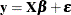This discussion parallels the discussion in Davidson and MacKinnon, 1993, pg. 548–562. The assumptions that make the linear regression best linear unbiased estimator (BLUE) are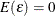and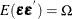, where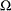has the simple structure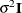. Heteroscedasticity results in a general covariance structure, so that it is not possible to simplify. The result is the following: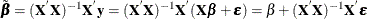As long as the following is true, then you are assured that the OLS estimate is consistent and unbiased: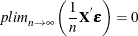If the regressors are nonrandom, then it is possible to write the variance of the estimatedas the following: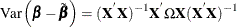The effect of structure in the variance-covariance matrix can be ameliorated by using generalized least squares (GLS), provided that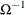can be calculated. Using, you premultiply both sides of the regression equation,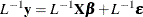where
denotes the Cholesky root of. (that is,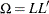withlower triangular).

The resulting GLSis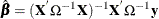Using the GLS, you can write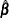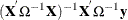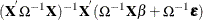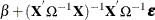The resulting variance expression for the GLS estimator is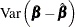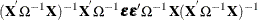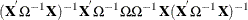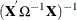The difference in variance between the OLS estimator and the GLS estimator can be written as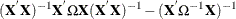By the Gauss-Markov theorem, the difference matrix must be positive definite under most circumstances (zero if OLS and GLS are the same, when the usual classical regression assumptions are met). Thus, OLS is not efficient under a general error structure. It is crucial to realize that OLS does not produce biased results. It would suffice if you had a method for estimating a consistent covariance matrix and you used the OLS. Estimation of thematrix is certainly not simple. The matrix is square and has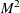elements; unless some sort of structure is assumed, it becomes an impossible problem to solve. However, the heteroscedasticity can have quite a general structure. White (1980) shows that it is not necessary to have a consistent estimate of. On the contrary, it suffices to calculate an estimate of the middle expression. That is, you need an estimate of: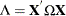This matrix,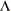, is easier to estimate because its dimension is K. PROC PANEL provides the following classical HCCME estimators for:

The matrix is approximated by:

• HCCME=N0: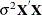This is the simple OLS estimator. If you do not specify the HCCME= option, PROC PANEL defaults to this estimator.

• HCCME=0: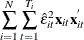where
is the number of cross sections and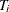is the number of observations inth cross section. The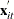is from theth observation in theth cross section, constituting the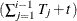th row of the matrix. If the CLUSTER option is specified, one extra term is added to the preceding equation so that the estimator of matrixis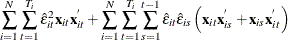• HCCME=1: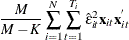where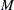is the total number of observations,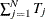, and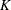is the number of parameters. With the CLUSTER option, the estimator becomes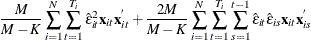• HCCME=2: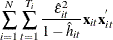The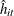term is theth diagonal element of the hat matrix. The expression foris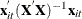. The hat matrix attempts to adjust the estimates for the presence of influence or leverage points. With the CLUSTER option, the estimator becomes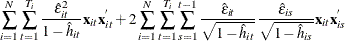• HCCME=3: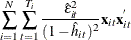With the CLUSTER option, the estimator becomes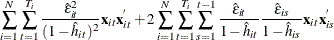• HCCME=4: PROC PANEL includes this option for the calculation of the Arellano (1987) version of the White (1980) HCCME in the panel setting. Arellano’s insight is that there arecovariance matrices in a panel, and each matrix corresponds to a cross section. Forming the White HCCME for each panel, you need to take only the average of thoseestimators that yield Arellano. The details of the estimation follow. First, you arrange the data such that the first cross section occupies the firstobservations. You treat the panels as separate regressions with the form: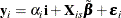The parameter estimates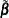and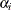are the result of least squares dummy variables (LSDV) or within estimator regressions, andis a vector of ones of length. The estimate of theth cross section’s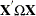matrix (where thesubscript indicates that no constant column has been suppressed to avoid confusion) is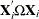. The estimate for the whole sample is: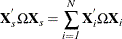The Arellano standard error is in fact a White-Newey-West estimator with constant and equal weight on each component. In the between estimators, selecting HCCME=4 returns the HCCME=0 result since there is no 'other' variable to group by.

In their discussion, Davidson and MacKinnon (1993, pg. 554) argue that HCCME=1 should always be preferred to HCCME=0. Although HCCME=3 is generally preferred to 2 and 2 is preferred to 1, the calculation of HCCME=1 is as simple as the calculation of HCCME=0. Therefore, it is clear that HCCME=1 is preferred when the calculation of the hat matrix is too tedious.

All HCCME estimators have well-defined asymptotic properties. The small sample properties are not well-known, and care must exercised when sample sizes are small.

The HCCME estimator of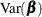is used to drive the covariance matrices for the fixed effects and the Lagrange multiplier standard errors. Robust estimates of the variance-covariance matrix forimply robust variance-covariance matrices for all other parameters.

 The dummy variables are removed by the within transformations, so their variances and covariances cannot be calculated the same way as the other regressors. They are recovered by the formulas listed in the sections One-Way Fixed-Effects Model and Two-Way Fixed-Effects Model. The formulas assume homoscedasticity, so they do not apply when HCCME is specified. Therefore, standard errors, variances, and covariances are reported only when the HCCME option is ignored. HCCME standard errors for dummy variables and intercept can be calculated by the dummy variable approach with the pooled model.